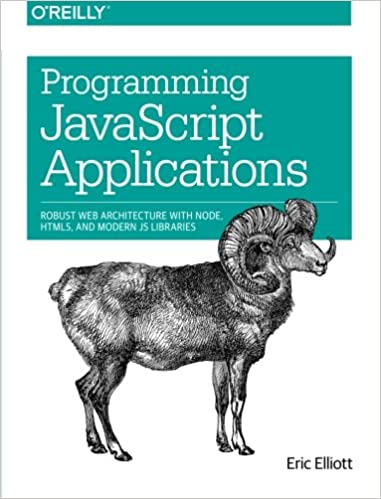Composing Software
Eric Elliott

### Why Funtional Programming?

Even if you don’t want to write a single line of code in what’s called funcitonal programming, it’s the mindset that matters. What makes functional programming a reliable mindset is that it’s based on several axioms that simplify how you think about your code.

#### Pure functions

• Same input gives the same output.
• A pure function doesn’t produce side effects. Which means it doesn’t modify any extrensic variables, doesn’t trigger external events, doesn’t produce any output to consoles / files / screens.
``````const add = (x, y) => {
return x + y
}
``````
``````const add = (x, y) => {
console.log('Hi!')
return x + y
}
``````

The first function adds two numbers and returns the summation. While the second function, adds two number, returns the summation, AND print out a message to the console. This will make the function impure since it does more than its job.

### Currying

Currying is a technique to convert function of multiple arugmnets to a sequence of functions that each takes a single argument at a time! That’s a lot – let’s see an example:

Without currying:

``````const add = (a, b) => a + b
add(2, 3) // return 5
``````

With currying:

``````const add = a => b =>  a + b
add(2)(3) // returns 5
``````

Currying comes handy when you want an argument to be fixed on function calling, which means to sepcialize the generic functionaly of a function to do specific job.

### Specialization and Generalization

There are two important parts of abstraction:

• Generalization The process of extracting only the shared properties and behaviors that serve the general use case
• Specialization The process of providing the implementation details required to serve the special case

Currying is probably used to specialize functions. From the above example, we can specialize the generic adding functionlity to make increment function by only 1:

``````const increment = add(1)
increment(2) //  => 3
``````

Another example is to specilize the module operation to find whether the number is odd or even:

``````const modulo = a => b => b % a // curried module
module(3)(5) // 5 % 3 => 2

const isOdd = modulo(2)

isOdd(2) // => 0
isOdd(3) // => 1
isOdd(4) // => 0
``````

This way we kept the `2` arugment fixed on every `isOdd` call.